# Summing Data from a Cell in a Number of Sheets in Microsoft Excel 2010

In this article, we will learn how to sum the data from a cell in a number of sheets.We use the Sum function with 3D reference in Microsoft Excel 2010.

SUM:This function is used to add the numbers in a range of cells.

The syntax of SUM formula:-  =SUM(number1,number2,……)

Let’s take an example to understand how we can add the numbers for rows.

We have sales data in range A1:A10. Now, we want to return the totalvalue.

• Select the cell A11.
• Write the formula.
• =SUM(A2:A10), and press Enter on your keyboard.
• The function will return the total value.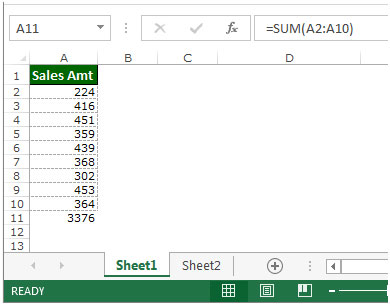Let’s take an example and understand how we can add the data values from the different sheets.

In this example, we have a workbook with four successive sheets: January, February, March and Total.

In every sheet, we have sales data month wise. Column A contains Agent name, column B contains country name and column C contains Sales amount.

In the total sheet, we want to return the total amount of every agent in Column C.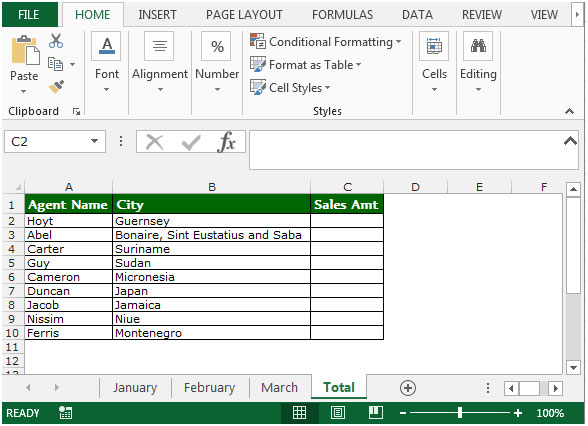• Select the cell C2 in Total’s sheet.
• Write the formula.
• =SUM(  , select the tab for the first sheet, January.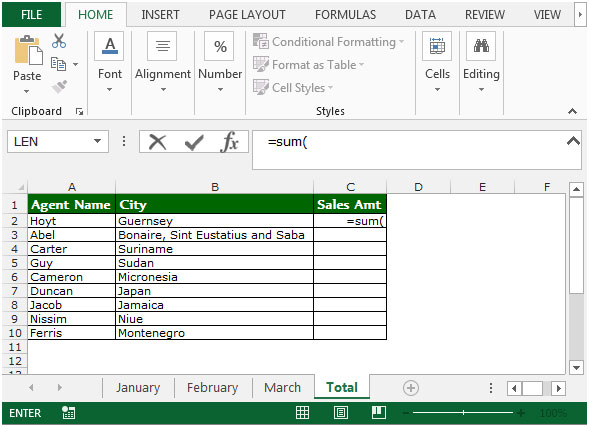• Hold the Shift key and then select the tab for the last sheet, March.
• Select cell B2, and then press Enter on your keyboard.
• The Formula is now =SUM(January:March!C2).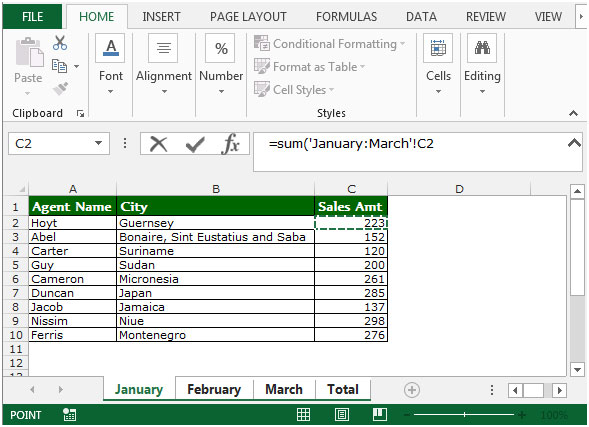• The function will return the total sales amount for HOYT in cell C2.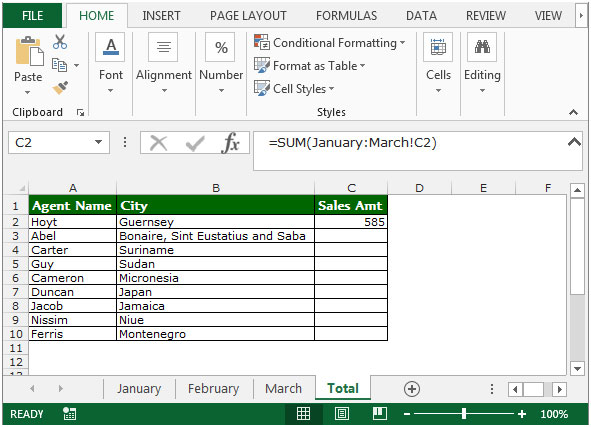• To return the total sales amount for every agent, copy the same formula by pressing the key Ctrl+C and paste in the range C3:C10 by pressing the key Ctrl+V on your keyboard.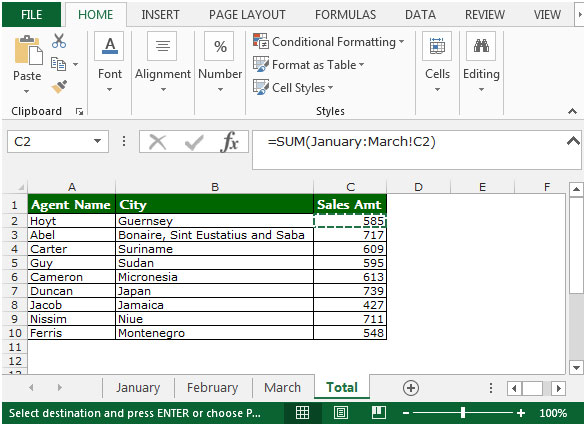This is the way you can get the sum of the data from a cell in a number of sheets in Microsoft Excel.

1.i have a question .. how will the sum know that it has to find the sum for Hoyt i dont see that in the sum equation

•Hi Krishna,

It is not finding HOYT in different sheets. It is already assumed that the HOYT comes in C2 of every sheet.

Terms and Conditions of use

The applications/code on this site are distributed as is and without warranties or liability. In no event shall the owner of the copyrights, or the authors of the applications/code be liable for any loss of profit, any problems or any damage resulting from the use or evaluation of the applications/code.